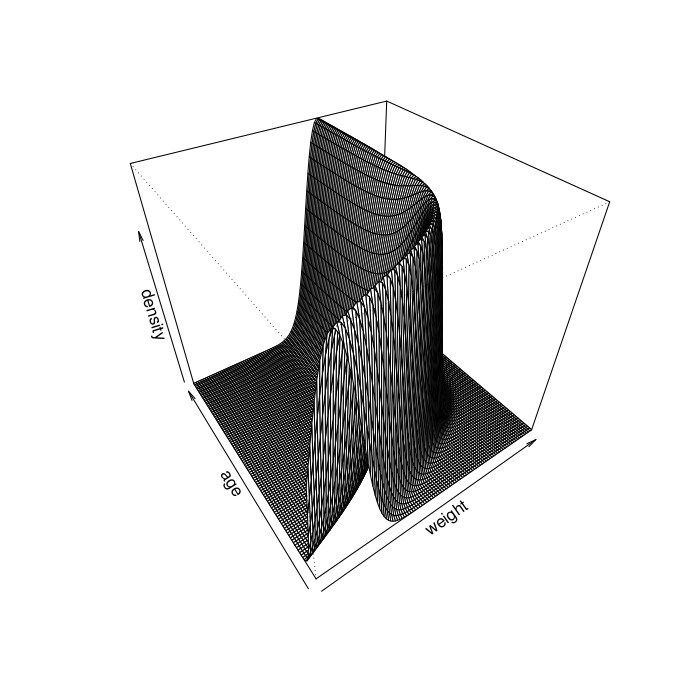Want to share your content on R-bloggers? click here if you have a blog, or here if you don't.

### Gompertz Model Visualization``` # Gomperz growth function gomp <- function(x, a, b, k) a*exp(-b*exp(-k*x))   # Normal model with Gompertz mean function likelihood <- function(weight, age, sigma, a, b, k) { mu <- gomp(age, a, b, k) dnorm(weight, mu, sigma) }   # Visualize the model visualize <- function(phi=40, theta=-35) { weight <- seq(0, 250, length.out=100) age <- seq(0, 50, length.out=100) dens <- outer(weight, age, likelihood, sigma=20, a=170, b=2, k=0.21) persp(weight, age, dens, phi=phi, theta=theta, xlab="weight", ylab="age", zlab="density") } ```

### Web Presentation for Data Frames

I know there is some functionality for this in the Hmisc and R2HTML packages. Can you get alternating row colors with the functions in these packages?

 Murder Assault UrbanPop Rape Alabama 13.2 236 58 21.2 Alaska 10 263 48 44.5 Arizona 8.1 294 80 31 Arkansas 8.8 190 50 19.5 California 9 276 91 40.6 Colorado 7.9 204 78 38.7
```   # Try: # data(USArrests) # webpage(head(USArrests))   webpage <- function(object, ...) UseMethod("webpage") HEADER <- " table { border: 0px; padding: 0px; } tr.even { background: #E2EBF0; text-align: right; } tr.odd { background: #FFFFFF; text-align: right; } tr.name { background: #1F2D49; color: white; text-align: center; } td.name { background: #1F2D49; color: white; text-align: left; } " FOOTER <- " "   webpage.data.frame <- function(object, header=HEADER, footer=FOOTER, ...) { esc <- function (text) { text <- gsub("&", "&", text) text <- gsub("\"", """, text) text <- gsub("'", "'", text) text <- gsub(">", ">", text) gsub("<", "<", text) } row_count <- nrow(object) col_count <- ncol(object) row_names <- gsub(" ", " ", row.names(object)) col_names <- gsub(" ", " ", names(object)) cat(header, "", sep="") evenodd <- "even" for(i in 1:row_count) { cat("", sep="") evenodd <- ifelse(evenodd=="even", "odd", "even") } cat("", paste(esc(col_names), collapse=""), "", esc(row_names[i]), "", paste(esc(format(object[i,], ...)), collapse = ""), "",footer) } ```

I was surprised to find little information regarding compressed file uploads in the PHP / JavaScript literature. The function below serves this purpose (but may not be fault tolerant). It would be cool to use this function in conjunction with a local HTTP server (running in R) to provide a web interface to compress and upload files to remote servers. This function assumes that the remote server has a mechanism to receive the data. I've included a server-side CGI shell script below that simply writes the (compressed) data to disk. Alternatively, one could set up a server-side R script, using rApache to simultaneously receive, decompress, and store the data.

``` # This function compresses a file using 'xz -9' compression # and uploads the file to a server using the HTTP POST method. # 'packpost' is shorthand for 'compress and upload'. The receiving # server should be set up to receive this upload using a server-side # scripting mechanism. packpost <- function(file, host="localhost", port="80", location="/", quiet = FALSE, query = URLencode(file)) {   if(!is.character(file) || length(file) != 1) stop("'file' must be a character vector of length 1") if(!is.character(host) || length(host) != 1) stop("'host' must be a character vector of length 1") if(!is.character(port) || length(port) != 1) stop("'port' must be a character vector of length 1") if(!is.character(location) || length(location) != 1) stop("'location' must be a character vector of length 1") if(!is.logical(quiet) || length(quiet) != 1) stop("'quiet' must be a logical vector of length 1") if(!is.character(query) || length(query) != 1) stop("'query' must be a character vector of length 1")   # pack cfile <- tempfile() fcon <- file(file, open="rb") ccon <- xzfile(cfile, open="wb", compression=9) if(!quiet) cat("packpost: compressing", file, "->", cfile, "\n") while(length(buff <- readBin(fcon, "raw", 1024)) > 0) writeBin(buff, ccon) close(fcon) close(ccon) if(!quiet) cat("packpost: compression ratio:", file.info(file)\$size / file.info(cfile)\$size, "\n")   # post if(!quiet) cat("packpost: uploading", cfile, "\n") location <- paste(URLencode(location), "?", URLencode(query), sep="") header <- paste("POST ", location, " HTTP/1.1\r\n", "Host: ", paste(host, port, sep=":"), "\r\n", "Content-Length: ", file.info(cfile)\$size, "\r\n", "Content-Type: application/x-xz\r\n\r\n", sep="")   ccon <- file(cfile, open="rb") scon <- socketConnection(host, port, open="w+b", blocking=TRUE) cat(header, file=scon) while(length(buff <- readBin(ccon, "raw", 1024)) > 0) writeBin(buff, scon) response <- readLines(scon, n=1) close(scon) close(ccon) if(!quiet) cat("packpost: removing", cfile, "\n") unlink(cfile) return(response) } ```
``` #!/bin/bash # This script would be located in a CGI directory on a remote host. # Note that this script alone may not be safe. In particular, The web # server should be configured to limit the upload size / prevent malicious # uploads. DATAFILE="upload-`date +%Y-%b-%d-%H%M-%N`"   # Append '.xz' when the data are xz compressed if [ "application/x-xz" = "\${CONTENT_TYPE}" ]; then DATAFILE="\${DATAFILE}.xz" fi   # POST data come from STDIN cat > \${DATAFILE}   # Return control to CGI handler echo -e "\r\n" ```